Empirical Asset Pricing using CRSP Dataset¶

Many books and papers explain empirical asset pricing such as CAPM and the Fama-French 3-factor model, but without working through the data first hand and replicating the results myself, it is difficult to build intuition about the subject.

In the below we work through the CRSP monthly dataset to get a real feel of how the data looks like, and also try to replicate some of the standard results in .

In :
import pandas as pd
import numpy as np
from   numpy.linalg import lstsq
import matplotlib.pyplot as plt
from   scipy.stats import describe, linregress
import functools
from   IPython.display import display, clear_output
from   numba import double, void, f4
from   numbapro import cuda
import itertools
import time
from mpl_toolkits.mplot3d import Axes3D

%matplotlib inline
plt.style.use(('bmh'))

In :
dataLoc  = '/Users/unclechu/Dropbox/Datasets/CRSP/CRSP_monthly.csv'
dataLoc2 = '/Users/unclechu/Dropbox/Datasets/CRSP/CRSP_Riskfree_1_Month.csv'

def crsp_riskfree():
riskfree = pd.read_csv ( dataLoc2, parse_dates=, infer_datetime_format=True )
riskfree.rename( columns = {'qdate':'date', 'ave_1':'RF1M' }, inplace=True )
riskfree['RF1M'] = pd.to_numeric( riskfree.RF1M, errors='coerce')
riskfree['RF1M'] = riskfree['RF1M'].apply( lambda x: (np.exp(30./365.*(x/100.)) - 1 ))
riskfree.set_index( 'date', inplace=True )
return riskfree

riskfree = crsp_riskfree() # load data for riskfree rate

Out:
date
1963-05-31 2.985 0.002436 2.934 35 19630705.4 3.053 3.043 3.033 90 19630829.4
1963-06-28 3.005 0.002456 2.965 34 19630801.4 3.043 3.033 3.022 90 19630926.4
1963-07-31 3.097 0.002515 3.016 36 19630905.4 3.329 3.319 3.309 92 19631031.4
1963-08-30 3.300 0.002687 3.229 34 19631003.4 3.452 3.437 3.421 91 19631129.4
1963-09-30 3.462 0.002825 3.401 31 19631031.4 3.473 3.442 3.411 94 19640102.4
In :
def excess_return_grouper( sub_df ):

idx = sub_df.index.labels
dt  = sub_df.index.levels[idx]

if dt.month == 1:
label = '%s-%s' %( dt.year - 1, 12 )
else:
label = '%s-%.2d' %( dt.year, dt.month-1)

if not pd.Timestamp(label) > riskfree.index.max():
rf_rate = riskfree.loc[label]['RF1M'].values
sub_df['XRET'] = sub_df.RET - rf_rate
else:
sub_df['XRET'] = 0.0

return sub_df

def crsp_stock_data():
df =pd.read_csv ( dataLoc, parse_dates=, infer_datetime_format=True )
df = df.query('SHRCD==10 or SHRCD==11').copy()
df['RET'] = pd.to_numeric( df.RET, errors='coerce')
df['RETX'] = pd.to_numeric( df.RETX, errors='coerce')
df.dropna(subset=['TICKER', 'RET', 'RETX'], inplace=True)

# Compute market capitalization. Note : The number of shares outstanding, in thousands, is given by the SHROUT
# field. The month end price of the stock is taken from the ALTPRC field. We define the market cap as the absolute
# value of the product of the SHROUT and the ALTPRC fields, divded by 1000. This gives market cap in millions. The
# absolutely value is taken because when there is no trading activity in a stock, CRSP reports the price as the
# negative of the average of the most recent bid and ask prices.
df['MktCap'] = np.abs( df.SHROUT * df.ALTPRC ) / 1000.0
df.set_index(['date', 'TICKER'], inplace=True)
df.sortlevel(0, inplace=True)

df = df.groupby(level='date').apply( excess_return_grouper )

return df

df = crsp_stock_data()

Out:
PERMNO SHRCD DLPDT DLSTCD DLRET PRC RET SHROUT ALTPRC ALTPRCDT RETX MktCap XRET
date TICKER
1963-06-28 A 10495 10.0 NaN NaN NaN 50.250 -0.042857 10715.0 50.250 19630628.0 -0.042857 538.428750 -0.045293
AA 24643 11.0 NaN NaN NaN 64.125 0.007859 21369.0 64.125 19630628.0 0.007859 1370.287125 0.005423
AAC 38877 10.0 NaN NaN NaN 11.875 0.000000 4591.0 11.875 19630628.0 -0.010417 54.518125 -0.002436
AAE 26227 10.0 NaN NaN NaN 23.375 -0.056368 2064.0 23.375 19630628.0 -0.060348 48.246000 -0.058804
AAP 28863 10.0 NaN NaN NaN 3.750 0.428571 932.0 3.750 19630628.0 0.428571 3.495000 0.426135

Cumulative Market Capitalization¶

We calculate the cumulative market capitalization of the CRSP universe for each month $t$.

In :
#calculate cumulative market cap
mktcap_ts = df.groupby(df.index.get_level_values(0))['MktCap'].sum() / 1000. # in billions

fig = plt.figure( figsize = ( 12, 6 ))
mktcap_ts.plot()
plt.title('Total market capitalization of CRSP universe. (Monthly data)')
plt.show()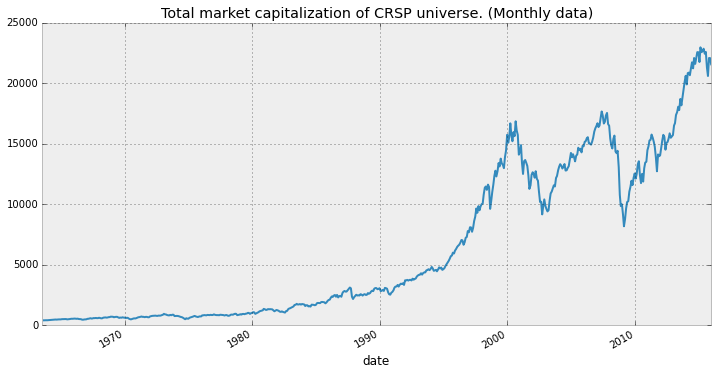Returns and Excess Returns for Cross-Section of CRSP Securities¶

We calculate returns and excess returns each month $t$ for the cross-section of stocks in the CRSP universe. From this we compute the cross-sectional means, variance, and other descriptive statistics for each time point t. Thus we end up with a time series of such values each month and we then summarise these time-series values.

In :
cx_excess_ret_info = df.groupby(level='date').apply( lambda x : x['XRET'].describe() )
cx_ret_info = df.groupby(level='date').apply( lambda x : x['RET'].describe())

for col in cx_excess_ret_info.columns:
if col != 'count':
cx_excess_ret_info[col] = cx_excess_ret_info[col] * 100
cx_ret_info[col] = cx_ret_info[col] * 100

In :
cx_excess_ret_info.head()

Out:
XRET count mean std min 25% 50% 75% max
date
1963-06-28 1973.0 -1.260115 8.210131 -75.243584 -5.082284 -1.758784 1.686916 99.756416
1963-07-31 1977.0 -0.895603 10.115783 -40.245644 -5.373844 -1.422144 1.928256 255.309956
1963-08-30 1978.0 3.970876 10.207320 -50.251494 -0.617769 3.138306 8.179056 214.034206
1963-09-30 1974.0 -1.360220 9.493721 -40.268717 -6.388517 -2.366617 1.654383 124.731283
1963-10-31 1976.0 0.977122 9.586472 -38.743980 -3.880005 -0.282480 4.116620 92.740820
In :
cx_ret_info.head()

Out:
RET count mean std min 25% 50% 75% max
date
1963-06-28 1973.0 -1.016531 8.210131 -75.0000 -4.838700 -1.5152 1.93050 100.0000
1963-07-31 1977.0 -0.649959 10.115783 -40.0000 -5.128200 -1.1765 2.17390 255.5556
1963-08-30 1978.0 4.222370 10.207320 -50.0000 -0.366275 3.3898 8.43055 214.2857
1963-09-30 1974.0 -1.091503 9.493721 -40.0000 -6.119800 -2.0979 1.92310 125.0000
1963-10-31 1976.0 1.259602 9.586472 -38.4615 -3.597525 0.0000 4.39910 93.0233
In :
ret_summary = pd.DataFrame([cx_excess_ret_info.apply(np.mean),
cx_ret_info.apply(np.mean)], index=['xret', 'ret'])
ret_summary

Out:
count mean std min 25% 50% 75% max
xret 4670.234548 0.823093 15.110622 -66.812508 -6.438214 -0.286295 6.372861 259.735219
ret 4670.234548 1.206966 15.405008 -67.864703 -6.154514 0.096216 6.846703 268.346641

From the above, we can see that the average cross-sectional return has a time-series mean of 1.2, and a value of 0.83 for excess returns. The min can be as low as more than -60% and the max as high as 250%. The illustrate the high risk of investing in stocks.

The Market Factor¶

The market factor is one of the import risk factors that features itself in the CAPM as well as the Fama-French 3-factor model. We use the market factor to compute the $\beta$ in the subsequent sections. Now we load and give a preview of the data.

In [ ]:
def FF_mkt_returns( daily=True ):
if daily:
dataLoc = '/Users/unclechu/Dropbox/Datasets/CRSP/FF-Factors-Daily.csv'
else:
dataLoc = '/Users/unclechu/Dropbox/Datasets/CRSP/FF-Factors-Monthly.csv'

df.set_index( 'dateff', inplace=True)
df.sort_index( inplace=True )
return df

mkt_returns = FF_mkt_returns(False)

In [ ]:
cum_xret = mkt_returns.mktrf + 1.
cum_xret = np.cumprod( cum_xret ) * 100.

fig = plt.figure( figsize = ( 12, 6))
cum_xret.plot()
plt.title('Cumulative excess return of the market portfolio')
plt.show()


Calculating Beta for the CRSP Universe ($\beta$)¶

The CAPM postulates that the excess return of asset $i$, $R^i$, can be explained by it's sensitivity to market risk $\beta_i$, via the following formulation,

\begin{aligned} &\mathbb{E}_t\left[R^i -R^f \right] = \beta_i\, \mathbb{E}_t\left[R^m -R^f \right] + (\alpha_i)\\ \Rightarrow &\mathbb{E}_t\left[R^{ei}\right] = \beta_i\, \mathbb{E}_t \left[R^{em}\right] + (\alpha_i) \end{aligned}

What CAPM theory says is that if a stock has high average returns, it should also have a high $\beta$ against average return of the market portfolio. Additionally, according to the CAPM, we should not see any $\alpha_i$. In reality, $\alpha_i$ is observed in practice and is statistically significant in some instances.

We derive $\beta_i$ from time-series regressions,

$$R^{ei}_t = \alpha_i + \beta_i R ^{em}_t + \epsilon^i_t$$

The point to stress about the above is that it's not about "predicting returns"! The whole point of the exercise is rather to see if high returns are matched by high $\beta_i$. From properties of least square regression we have the following,

1. $\beta_i$ is given by $\beta_i = \frac{\text{cov}(R^{ei},R^{em})}{\text{var}(R^{em})}$
2. $\text{cov}(\epsilon^i, R^{em}) = 0$

We calculate the cross-sectional values of beta across the entire stock universe to gain some intuition on the range of values of $\beta$ and it's behavior over time. To attack this huge computational task, we use the GPU to perform the OLS regressions in parallel.

In :
@cuda.jit( void( double[:,:], double[:,:], double[:,:] ))
def ols( x, y, ret ):
''' batch compute large number of least sqaure regression y = a + bx '''
i = cuda.grid(1)
N = y.shape

xbar = 0.0
ybar = 0.0
for k in range(N):
xbar += x[i][k]
ybar += y[i][k]

xbar = xbar / N
ybar = ybar / N

nom = 0.0
denom = 0.0

for k in range(N):
nom += ( x[i][k] - xbar ) * ( y[i][k] - ybar)
denom += ( x[i][k] - xbar )**2

beta = nom / denom
ret[i] = beta
ret[i] = ybar - beta * xbar

def chunk_iter( iterable, n ):
it = iter(iterable)
while True:
chunk = tuple(itertools.islice(it, n))
if not chunk:
return
yield chunk

def batch_ols( xlist, ylist, tickers, dt ):

stream = cuda.stream()
griddim = 1, 1

xs = np.stack( xlist )
ys = np.stack( ylist )
cnt = len( ylist )
ret = np.zeros( ( cnt, 2 ) )
blockdim = cnt, 1, 1

dxs  = cuda.to_device( xs, stream )
dys  = cuda.to_device( ys, stream )
dret = cuda.to_device( ret, stream )

ols[griddim, blockdim, stream](dxs, dys, dret)
dret.to_host(stream)
stream.synchronize()

alpha, beta = zip(*ret)
df = pd.DataFrame.from_dict( dict(ticker=tickers, alpha=alpha, beta=beta ) )
df['date'] = dt

return df

class Timer():

def __init__( self, desc ):
self.desc = desc

def __enter__( self ):
self.start = time.time()
return self

def __exit__(self, type, value, traceback):
self.elapsed = time.time() - self.start
print self.desc, 'took', self.elapsed, 'seconds'


In :
def do_beta():

CHUNK_SIZE =  1024 #1536
monthly_data_points = 24 # number of points we use to compute beta.

stock_data = crsp_stock_data()
riskfree = crsp_riskfree()
mkt_returns = FF_mkt_returns(daily=False)

stock_data['XRET'] = 0.0

# compute excess returns for each month across the whole dataset
for dt in riskfree.index[1:]:

if dt.month == 1:
prev_month = '%s-%.2d' % (dt.year-1,12)
else:
prev_month = '%s-%.2d' % (dt.year,dt.month-1)

riskfree_rate = riskfree.loc[prev_month]['RF1M'].values # only 1 row in the series, pick out the atom value
stock_data.loc[(dt, slice(None)), 'XRET'] = stock_data.loc[(dt, slice(None)), 'RET'] - riskfree_rate

result_dfs = []
for dt in riskfree.index[monthly_data_points:]:
clear_output(wait=True)
display( 'processing %s' % dt.date() )

start_date = dt + pd.Timedelta(days=-365*2)
end_date   = dt

period_mkt_returns = mkt_returns.ix[start_date:end_date]
stock_data_window = stock_data.loc[ start_date : end_date ]
tickers = stock_data_window.loc[dt].index.unique()

count = 0
for chunk in chunk_iter( tickers, CHUNK_SIZE ):
# prepare the data for execution on the GPU
symbols, xlist, ylist = [], [], []

for ticker in chunk:
if not isinstance(ticker, str):
continue

ticker_df = stock_data_window.loc(axis=0)[:,ticker]

if all( np.isfinite( ticker_df.RET ) ):

# take data point for the last 24 months
y = ticker_df[-24:]['XRET']
x = period_mkt_returns[-24:]['mktrf']
if not ( len(x) == 24 and len(y) == 24 ):
continue

ylist.append(y.values)
xlist.append(x.values)
symbols.append(ticker)

# send everything to the GPU
if len( xlist ) > 0 and len( ylist ) > 0:
cur_df = batch_ols( xlist, ylist, symbols, dt.date() )
result_dfs.append(cur_df)

return result_dfs

In [ ]:
# compute all the beta values if this is first run. This takes ~2hrs
# otherwise load existing computed date from disk.
with Timer('Computing beta') as t:
beta_data = do_beta()
beta_df = pd.concat(beta_data, ignore_index=True)
beta_df.set_index(['date', 'ticker'], inplace=True)
beta_df.sort_index(0, inplace=True)

In :
# read betas from what we've already computed and saved previously.
parse_dates=, infer_datetime_format=True)
beta_df.set_index(['date', 'ticker'], inplace=True)
beta_df.sort_index(0, inplace=True)


We show a plot of time-series of CAPM beta for selected stocks from the CRSP universe. Note the fluctuating behavior of $\beta$ through time for each of the stocks.

In :
fig = plt.figure( figsize = ( 12, 6))
symbols = ['AAPL', 'MSFT', 'XOM', 'DIS']
for sym in symbols:
beta = beta_df.loc[ (slice(None), sym), ][['beta']]
plt.plot(beta.index.get_level_values(0), beta.beta, label = sym)

plt.title('Calculated values of CAPM $\\beta$ for %s' % ['AAPL', 'MSFT', 'XOM', 'DIS'])
plt.legend()
plt.show()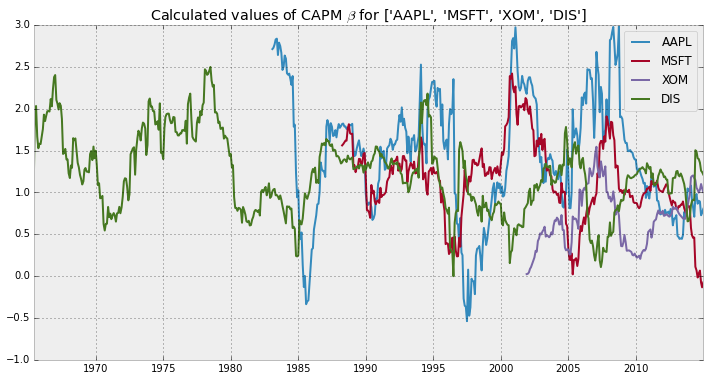We replicate below the same results as in  for the summary statistics of the cross-sectional measurement of beta for months t from 1962 to 2014. The symbol $\beta^{2Y}$ refers to the beta value computed using 2 years of monthly returns data for each month $t$.

In :
def beta_group_func( sub_df ):
return sub_df.beta.describe()

beta_info = beta_df.groupby(beta_df.index.get_level_values(0)).apply(beta_group_func)

beta_summary = pd.DataFrame([beta_info.apply(np.mean)], index=['$\\beta^{2Y}$'])
beta_summary

Out:
beta count mean std min 25% 50% 75% max
$\beta^{2Y}$ 3698.122689 1.132501 0.927334 -4.31045 0.548272 1.032542 1.615988 8.922931

Cross-Section Visualization of $\beta$ over time¶

We next show a 3D histogram of the distribution of the cross-sectional values of beta over time. This gives us greater intuition of the distributional characteristics of $\beta$ through time.

In :
import matplotlib.colors as colors
import matplotlib.cm as cmx

bins = np.linspace(-10, 10, 50)
dateHistPairs = []

for dt, sub_df in beta_df.groupby( beta_df.index.get_level_values(0)):
betas = sub_df['beta']
hist, _ = np.histogram( betas, bins=bins )
dateHistPairs.append( ( dt, hist ))

In :
N=50
data = dateHistPairs[-N:]

fig = plt.figure(figsize=(16,12))

cm = plt.get_cmap('Paired')
vv = range(len(data))
cNorm = colors.Normalize(vmin=0, vmax=N)
scalarMap = cmx.ScalarMappable(norm=cNorm, cmap=cm)
colorVals = [scalarMap.to_rgba(i % N) for i in range(len(data))]

for i, (dt, hist) in enumerate( data ):
xpos = bins[:-1]
ypos = np.ones_like(xpos) * i * 0.25
zpos = np.zeros_like(xpos)
dx = ( bins - bins - 0.1) * np.ones_like(xpos)
dy = 0.25 * np.ones_like(xpos)
dz = hist
ax.bar3d(xpos, ypos, zpos, dx, dy, dz, zsort='max', color=colorVals[i ])

ys      = np.array(range(len(data))) * 0.25
ylabels = beta_df.index.get_level_values(0).unique()[-len(data):]
ylabels = [str(x.date()) for x in ylabels]

plt.yticks(ys[::4], ylabels[::4], rotation=-15)

for label in ax.yaxis.get_ticklabels():
label.set_horizontalalignment('left')
label.set_verticalalignment('bottom')

plt.ylim([-0.5, len(data) * 0.25 + 0.5])
plt.title('Plot of monthly histogram of the distribution of $\\beta$ through time.')
plt.show()

/Users/unclechu/anaconda/lib/python2.7/site-packages/matplotlib/collections.py:590: FutureWarning: elementwise comparison failed; returning scalar instead, but in the future will perform elementwise comparison
if self._edgecolors == str('face'):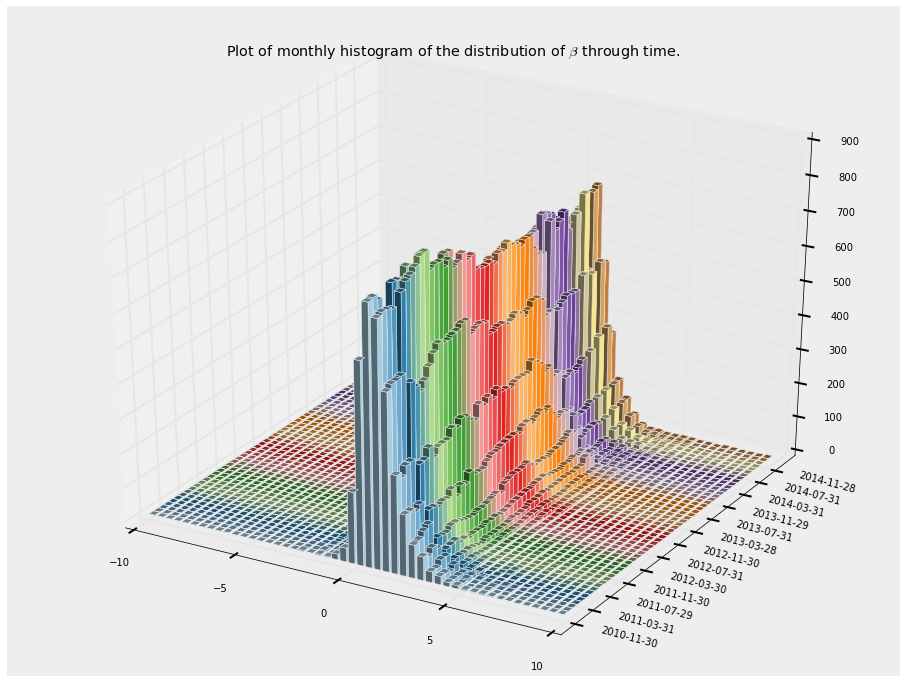As we can see from above, the distribution of the values of $\beta$ through time it's quite stable and mainly falls in the range of $-0.1$ to $5.0$.

Decile Portfolios sorted on $\beta$¶

We now investigate the excess returns on decile portfolios sorted on $\beta$. In each month $t$, we sort the CRSP universe according their computed values of beta $\beta^{2Y}$.

In :
decile_labels = [ 'D%.2d' % x for x in range(1, 11 )]

def decile_portfolios( beta_df_month_t ):
''' sort the stocks into 10 decile portfolios based on the beta values '''
beta_df_month_t['quantile'] = pd.qcut(beta_df_month_t.beta, 10, labels=decile_labels)
return beta_df_month_t

quantiled_beta = beta_df.groupby(beta_df.index.get_level_values(0)).apply( decile_portfolios )

Out:
alpha beta quantile
date ticker
1965-05-28 A -0.005877 2.238277 D09
AA 0.001422 0.717909 D04
AAC -0.003686 0.637556 D04
AAE -0.000689 0.737196 D05
AAP -0.002193 0.502662 D04

With the above, we now have a quantile column indicating for each date which decile portfolio a ticker belongs to. We can now proceed to compute, for each month $t$, the returns on equal-weighted (or value-weighted) decile portfolios.

The betas calculated for month t are used to construct the 10 decile portfolios. The portfolios are then held from month t to month t+1. So the holding period return for month t+1 are the ones we use to calculate returns on the decile portfolios. In the below, we roll the dates for the beta dataframe one month forward, then perform a join with the securities dataframe to get all the data lined up so we can calculate the decile portfolio returns for each month t.

In :
# roll the dates one month forward for the beta dataframe
quantiled_beta.reset_index(inplace=True)
df.reset_index(inplace=True)

beta_datelist = quantiled_beta.date.unique()
maxdate = beta_datelist.max()

mapping = dict( zip( beta_datelist, np.roll(beta_datelist, -1)))

stockdates = df.date.unique()
max_plus_one = stockdates[stockdates > maxdate]

mapping[maxdate] = np.datetime64( max_plus_one )

# this because it's a nightmare working with datetimes in pandas
mapping = dict( ( pd.Timestamp(k).date(), pd.Timestamp(v).date() ) for k, v in mapping.items() )

quantiled_beta['date'] = pd.to_datetime( pd.Series( [ mapping[dt.date()] for dt in quantiled_beta['date'] ] ) )


Equal-Weighted Decile Portfolios sorted on $\beta$¶

In :
def decile_return_group_func( sub_df ):
return sub_df.groupby('quantile').apply( lambda q : q['XRET'].mean() * 100. )

m = pd.merge( df, quantiled_beta, left_on=['date','TICKER'], right_on=['date', 'ticker'])
decile_returns = m.groupby('date').apply( decile_return_group_func )
decile_returns['D10-D01'] = decile_returns.D10 - decile_returns.D01
decile_returns.describe()

Out:
quantile D01 D02 D03 D04 D05 D06 D07 D08 D09 D10 D10-D01
count 595.000000 595.000000 595.000000 595.000000 595.000000 595.000000 595.000000 595.000000 595.000000 595.000000 595.000000
mean 0.886820 0.802384 0.864926 0.926494 1.005636 0.994366 1.032051 0.960155 0.945973 0.846422 -0.040399
std 4.990144 4.307412 4.556105 4.950849 5.253391 5.741480 6.278537 6.929237 7.870522 9.612178 6.249384
min -26.323072 -24.625004 -25.318127 -24.391549 -26.906956 -27.465403 -28.860454 -29.567636 -30.924752 -33.503546 -25.718012
25% -1.714164 -1.437185 -1.496972 -1.847213 -1.866481 -2.107028 -2.483724 -3.133848 -3.683225 -4.750835 -3.416424
50% 0.937649 0.952040 1.038075 1.085078 1.204315 0.979290 1.238805 1.159444 1.075538 0.678310 -0.315549
75% 3.665313 3.367844 3.592294 3.862176 3.965993 4.398687 4.653883 5.020951 5.663000 6.080321 2.885052
max 23.244769 25.113244 28.590616 30.679661 29.420248 29.634953 31.058783 30.704531 39.459343 54.313095 42.353782

From the above results, we can see that clearly the claim that higher returns correspond to higher betas is clearly not true. In fact, the return on the decile-10 portfolio minus the decile-1 portfolio is actually negative!.

In :
def decile_beta_group_func( sub_df ):
return sub_df.groupby('quantile').apply( lambda q : q['beta'].mean() )

decile_betas = m.groupby('date').apply( decile_beta_group_func )
decile_betas

fig = plt.figure( figsize = ( 12, 6))

x = decile_betas.apply( np.mean )
y = decile_returns.apply( np.mean )[:-1]
A = np.vstack([x, np.ones(len(x))]).T
b, c = np.linalg.lstsq(A, y)

plt.plot(x, b*x + c, 'black', label='Fitted line')
plt.scatter( x, y, marker='^', s=150, c='pink', edgecolors='black', linewidths=2.0)

plt.title('Graph of $\\beta$ against average excess returns for the 10 decile portfolios (equal-weighted)')
plt.legend()
plt.show()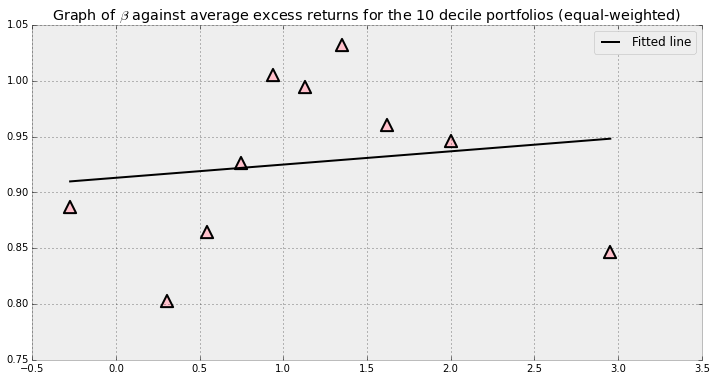The graph above presents the results more clearly. As we can see, the betas do not line up ( as they should be according to the CAPM ).

Value-Weighted Decile Portfolios sorted on $\beta$¶

We next show similar analysis as the above for value-weight decile portfolios. The market-capitalization for each stock is used as the weights.

In :
def decile_return_group_func( sub_df ):
return sub_df.groupby('quantile').apply( lambda q : np.average(q['XRET'], weights=q['MktCap']) * 100. )

m = pd.merge( df, quantiled_beta, left_on=['date','TICKER'], right_on=['date', 'ticker'])
decile_returns = m.groupby('date').apply( decile_return_group_func )
decile_returns['D10-D01'] = decile_returns.D10 - decile_returns.D01
decile_returns.describe()

Out:
quantile D01 D02 D03 D04 D05 D06 D07 D08 D09 D10 D10-D01
count 595.000000 595.000000 595.000000 595.000000 595.000000 595.000000 595.000000 595.000000 595.000000 595.000000 595.000000
mean 1.096408 0.866639 0.975160 1.128773 1.078564 1.095950 1.232284 1.312247 1.623345 2.118625 1.022217
std 4.155015 3.996773 3.958069 4.158769 4.478403 4.984347 5.531112 6.110282 7.406259 9.300748 7.874649
min -26.798936 -23.182969 -19.663387 -15.706277 -16.581529 -19.703912 -22.006420 -24.472719 -25.655322 -32.991885 -34.087267
25% -1.197033 -1.180849 -1.435307 -1.441181 -1.614884 -2.038471 -2.077860 -2.409929 -2.816007 -3.811308 -3.664297
50% 1.105593 0.902338 1.123150 1.187471 1.305843 1.248619 1.516560 1.336603 1.671202 1.860759 0.375854
75% 3.573948 3.086854 3.354536 3.782701 3.950937 4.226681 4.679214 4.979906 6.026512 7.433997 4.719352
max 21.847318 22.357143 17.582446 21.092909 19.096643 16.914534 21.343969 24.920978 35.756028 46.094523 39.598782

Contrary to the results for the equal-weight decile portfolios, the results for the value-weighted portfolios do show a slight positive return from being long the 10th decile portfolio and short the 1st decile portfolio.

In :
fig = plt.figure( figsize = ( 12, 6))

x = decile_betas.apply( np.mean )
y = decile_returns.apply( np.mean )[:-1]
A = np.vstack([x, np.ones(len(x))]).T
b, c = np.linalg.lstsq(A, y)

plt.plot(x, b*x + c, 'black', label='Fitted line')
plt.scatter( x, y, marker='^', s=150, c='pink', edgecolors='black', linewidths=2.0)

plt.title('Graph of $\\beta$ against average excess returns for the 10 decile portfolios (value-weighted)')
plt.legend()
plt.show()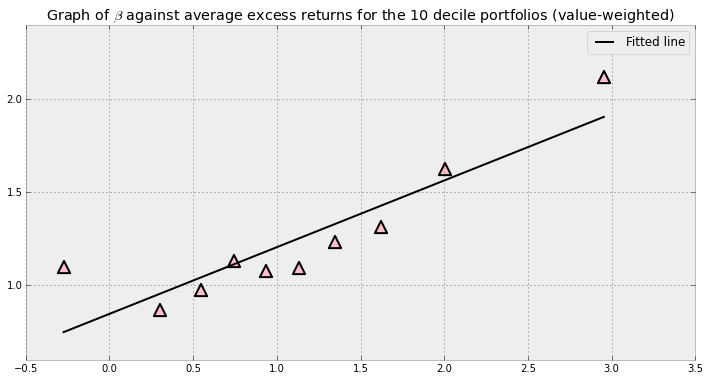As we can see, the value-weight portfolios behave much better, but there are still anomalies (outliers too far above the line ).

 Turan G. Bali, Robert F. Engle, Scott Murray, Empirical Asset Pricing: The Cross Section of Stock Returns. Wiley Series in Probability and Statistics, April 4, 2016.